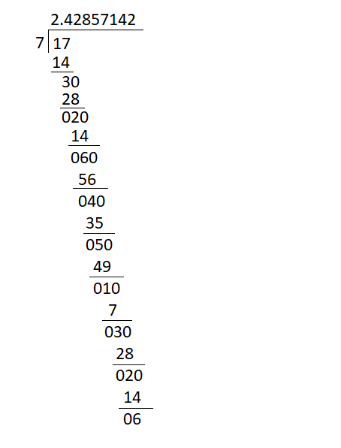# How many digits are there in the repeating block of digits in the decimal expansion of

Question:

How many digits are there in the repeating block of digits in the decimal expansion of $\frac{17}{7} ?$

(a) 16

(b) 6

(c) 26

(d) 7

Solution:$\because \frac{17}{7}=2 . \overline{428571}$

So, there are 6 digits in the repeating block of digits in the decimal expansion of $\frac{17}{7}$.

Hence, the correct option is (b).